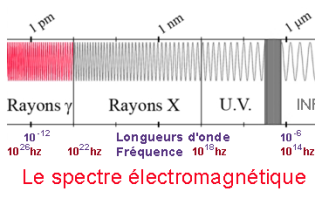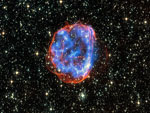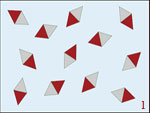# What is a wave?

## A wave is an energy that spreadsAutomatic translation Category: matter and particles
Updated July 25, 2014

Say that a wave is the propagation of a disturbance of the environment is not enough to understand what a wave.
Say that speed, wavelength and frequency are the three properties characterizing a wave and that the relation between the three variables is v = λ * ν that does not sufficient to understand what a wave.
Say that a wave travels with a specific speed which depends on the characteristics of the propagation environment, it does not show the wave.
Say that a wave carries energy without transporting of matter, it tells us, always nothing in the nature of a wave.
Now imagine a rope that is shaken, of a vertical gesture. This simple movement will draw a wave of a certain height, which appears to be spreading to the front but in reality no point in the rope moves each point, comes and goes, moving vertically. This amplitude gives us an idea of the force with which you shake the rope but not the wave itself.
To understand what is a wave must remove the rope and imagine the air molecules undergoing the mechanical pressure of the rope. At this point it only remains in the medium (air) than the energy, a force generated by the movement of the rope. This energy makes the pressure of the air oscillate around an equilibrium value, the pressure increases and decreases alternately around this value. The wave (energy) moves the air molecules without transport them. As the rope is shaken, one can measure the distance between two bosses or, two hollow, this distance is the wavelength, measured in meters. The rate at which the rope is shaken, corresponds to the wave frequency measured in Hertz. The wave velocity measured in meters / second is equal to the wavelength multiplied by frequency.

One wave propagates in a stable environment, able to return to an equilibrium state, for a sound wave that is the air pressure which moves relative to an average value, for an electromagnetic wave is the intensity of the electromagnetic field which moves relative to an average value of the field.
Electricity can be static, such as amber after being rubbed, attracts small objects. Magnetism can also be static like a magnet. But when these fields move together, they become electromagnetic waves (see image). Electromagnetic waves are formed when an electric field (shown by red arrows) couples with a magnetic field (represented by blue arrows). The electric and magnetic fields of an electromagnetic wave are always perpendicular one with respect to another but also perpendicular to the direction of the wave.
These two types of waves, mechanical and electromagnetic, are two ways of transporting energy in a medium. The waves in the water and the sound waves in the air are two examples of mechanical waves. This energy transport disrupts or makes vibrate matter (solid, liquid, gas or plasma) without transport, water or air molecules collide but remain in the same place.
A variable magnetic field induces a variable electric field and vice versa, the two are connected. When these two fields are coupled (Maxwell equations) form electromagnetic waves (see image). Electromagnetic waves unlike mechanical waves, do not need medium to propagate, they travel everywhere, even in the vacuum of space.
Light, electromagnetic waves and all radiation are from the same physical phenomenon: the electromagnetic energy.### Image: Electromagnetic waves are formed by the sinusoidal vibration of electric and magnetic fields. These fields are always perpendicular one with respect to another but also perpendicular to the direction of movement of the wave. Once formed, this energy travels at the speed of light until the next interaction with matter. An electromagnetic wave is a transverse wave.

nota: The wave is said transversal, if the energy moves perpendicularly to the direction of wave movement (movement of the arm, shaking the rope or the energy of a rock falling into the water). The wave is called longitudinal, if the energy moves in the direction of travel of the wave (the magnet of the speaker). A wave can be both longitudinal and transversal (a baguette, which strikes a drum).

## Frequency, wavelength and energy

Frequency, wavelength and energy are mathematically related, just know one of these three values ​​to calculate the other two. The microwaves and radio waves are generally described in terms of frequency (Hertz), infrared and visible light in terms of wavelength (λ), and x-rays and gamma rays in terms of energy (electron volts).
The wave frequency is the number of periodic phenomena (ridges) that breed in a second, it is given in Hertz, after Heinrich Hertz (1857-1894) that established the existence of radio waves. From radio waves to gamma rays, the frequency is measured a few Hertz to 1026 Hertz.
The wavelength is the distance between two peaks (the electromagnetic waves have crests and troughs similar to those of ocean waves). The longest waves (radio waves) can measure a few kilometers or more, while the shorter wavelength (gamma waves) produced by the atomic nucleus, can measure up to 10-12 m (size of the atomic nucleus).
The energy of an electromagnetic wave is measured in electron volts (eV). An electron-volt is the amount of kinetic energy required to move an electron through a voltage potential of 1 volt. The lowest energy is the energy of radio waves (few eV), while the highest energies are those gamma rays. Gamma rays are radiation, high-energy photons (above 100 keV) sufficient to remove an electron from its orbit. Gamma rays are the most energetic form of light.

nota: Between the wavelength (λ) and frequency (ν) is the following relationship: ν = c / λ
ν = wave frequency in hertz
c = speed of light in vacuum in m / s
λ = wavelength in meter1997 © Astronoo.com − Astronomy, Astrophysics, Evolution and Earth science. Contact    Mentions légalesScale of the nanoparticles...2015 International
year of light...Neutrino
and beta emission...Magnetic order
and magnetization...